# Grade - examples - page 65

1. Painting rooms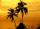If Dano paint three hours daily given work he complete in 7.5 days. How many hours a day would have to work to finish the job 1.5 days earlier?
2. Line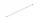How many parts of line divide 5 (different) points that lie on it?
3. Tractors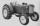Uncle is going buy tractor Zetor super 50. The first costs 81000 Eur, the second costs 98000 Eur. How much pay uncle for both tractors?
4. The hallThe hall had a rectangular ground plan one dimension 20 m longer than the other. After rebuilding the length of the hall declined by 5 m and the width has increased by 10 m. Floor area increased by 300 m2. What were the original dimensions of the hall?
5. Florist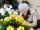Florist has 84 red and 48 white roses. How many same bouquets can he make from them if he must use all roses?
6. Landlord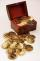Landlord had 49 ducats more than Jurošík. How many ducats Jurošík steal landlord if the Jurošík now 5 ducats more?
7. Diagonal in rectangle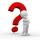In that rectangle ABCD is the center of BC point E and point F is center of CD. Prove that the lines AE and AF divide diagonal BD into three equal parts.
8. Copiers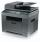The new copier copying a folder of papers 5 min. faster than the old. The operator used new, but out of toner and exchange took 5 min. In that time copied on the old. The whole work has been done for 9 min. How long would the work done only by old copier?
9. Homework - jumps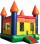Sasha jumped 121 cm Peter 4 cm more than Jirka. Jirka 6 cm less than Misha. Misha 7 cm less than Filip and Filip half as much Sasha and Petr together. How far each jumped?
10. Rectangle - sidesWhat is the perimeter of a rectangle with area 266 cm2 if length of the shorter side is 5 cm shorter than the length of the longer side?
11. FractionFraction ? write as fraction a/b, a, b is integers numerator/denominator.
12. Trousers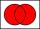In the class was 12 students. Nine students wearing trousers and turtleneck eight. How many students worn trousers with a turtleneck?
13. Angles and sides of the triangle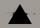Triangle ABC has a circumference of 26 cm. Lengths of the sides are as follows: a = 11.2 cm; b = 6.5 cm. Arrange the interior angles in order of its size. ?
14. Eight blocks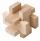Dana had the task to save the eight blocks of these rules: 1. Between two red cubes must be a different color. 2. Between two blue must be two different colors. 3. Between two green must be three different colors. 4. Between two yellow blocks must be four
15. Tachometer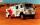Tatra's tachometer shows the initial state 886123 km this morning. Tatra today travel at an average speed of 44 km/h. Determine the function that describes the Tatra's tachometer depending on the time. What is the state of tachometer after 4 hours?
16. Bearing - navigationA ship travels 84 km on a bearing of 17°, and then travels on a bearing of 107° for 135 km. Find the distance of the end of the trip from the starting point, to the nearest kilometer.
17. Alloy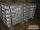The first alloy is a mixture of two metals in the ratio 1:2, the second is a mixture of same metals in the ratio 2:3. At what ratio we have these two alloys put into the furnace to obtain a new metal alloy with ratio 17:27? (All three ratios correspond to.
18. Parallelogram - sideCalculate the length of the side of a parallelogram whose area is 175 dm2 and height to that side is 24.2 dm long.
19. Pytagoriade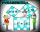Two fifth-graders teams competing in math competitions - in Mathematical Olympiad and Pytagoriade. Of the 33 students competed in at least one of the contest 22 students. Students who competed only in Pytagoriade was twice more than those who just competed
20. See harmonics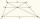It is true that the size of the central segment of any trapezoid is the harmonic mean size of its bases? Prove it. Central segment crosses the intersection of the diagonals and is parallel to the bases.

Do you have an interesting mathematical example that you can't solve it? Enter it, and we can try to solve it.

To this e-mail address, we will reply solution; solved examples are also published here. Please enter e-mail correctly and check whether you don't have a full mailbox.